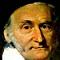# Math Is Fun Forum

Discussion about math, puzzles, games and fun.   Useful symbols: ÷ × ½ √ ∞ ≠ ≤ ≥ ≈ ⇒ ± ∈ Δ θ ∴ ∑ ∫  π  -¹ ² ³ °

You are not logged in.

## #1 2011-06-29 17:05:07

1a2b3c2212
Member
Registered: 2009-04-04
Posts: 419

### Functions and Relations

Suppose p is an integer such that p≥0 and q is a natural number. It is also defined that p mod q is the remainder when p is divided by q. For example, 4mod4= 0, 7mod4=3, 3mod4=3.

Given that A={0,1,2,3,4} and f:A->A is defined by f(x)=3x mod5.

a)Express the function f as a set of ordered pairs.
b)Find the range of f.
c) Explain whether f^-1 exists or not and find f^-1 if it exists.

Last edited by 1a2b3c2212 (2011-06-29 17:38:59)

Offline

## #2 2011-06-29 17:16:14

bobbym
bumpkinFrom: Bumpkinland
Registered: 2009-04-12
Posts: 109,606

### Re: Functions and Relations

Hi;

4mod4= 0, 7mod4=0, 3mod4=0.

The last 2 are not correct.  7 mod 4 = 3 and 3 mod 4 = 3.

In mathematics, you don't understand things. You just get used to them.
If it ain't broke, fix it until it is.
Always satisfy the Prime Directive of getting the right answer above all else.

Offline

## #3 2011-06-29 17:38:42

1a2b3c2212
Member
Registered: 2009-04-04
Posts: 419

woops typo

Offline

## #4 2011-06-29 22:53:41

gnitsuk
MemberRegistered: 2006-02-09
Posts: 121

### Re: Functions and Relations

1) {(0,0),(1,3),(2,1),(3,4),(4,2)}

2) From above the range of f(x) is A. This is because the value of f(x) can take any of all of the values from A = {0,1,2,3,4}.

3)

You can verify this by multiplying each second element in every ordered pair by 2 and reducing modulo 5 and seeing that the answer in each case is the first element in the corresponding ordered pair.

2 is said to be the multiplicative inverse of 3 mod(5)

Offline